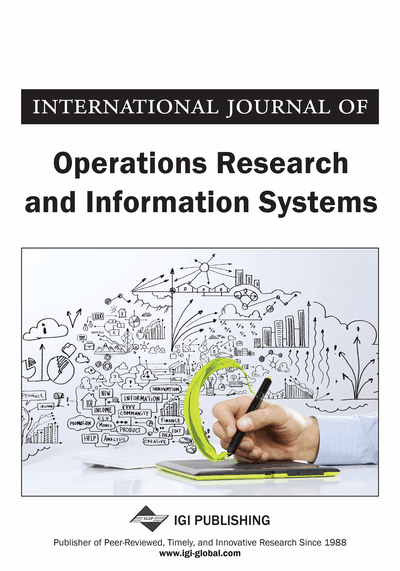# A Simple Method for Solving Fully Intuitionistic Fuzzy Real Life Assignment Problem

P. Senthil Kumar (PG & Research Department of Mathematics, Jamal Mohamed College (Autonomous), Tiruchirappalli, Tamil Nadu, India) and R. Jahir Hussain (PG & Research Department of Mathematics, Jamal Mohamed College (Autonomous), Tiruchirappalli, Tamil Nadu, India)
DOI: 10.4018/IJORIS.2016040103
Available
\$33.75
List Price: \$37.50
10% Discount:-\$3.75
TOTAL SAVINGS: \$3.75

## Abstract

In solving real life assignment problem we often face the state of uncertainty as well as hesitation due to various uncontrollable factors. To deal with uncertainty and hesitation many authors have suggested the intuitionistic fuzzy representations for the data. So, in this paper, the authors consider the assignment problem having uncertainty and hesitation in cost/time/profit. They formulate the problem and utilize triangular intuitionistic fuzzy numbers (TIFNs) to deal with uncertainty and hesitation. The authors propose a new method called PSK (P.Senthil Kumar) method for finding the intuitionistic fuzzy optimal cost/time/profit for fully intuitionistic fuzzy assignment problem (FIFAP). The proposed method gives the optimal object value in terms of TIFN. The main advantage of this method is computationally very simple, easy to understand. Finally the effectiveness of the proposed method is illustrated by means of a numerical example which is followed by graphical representation of the finding.
Article Preview
Top

## 1. Introduction

Assignment Problem (AP) is used worldwide in solving real world problems. An assignment problem plays an important role in assigning of persons to jobs, drivers to trucks, trucks to routes, operators to machines, or problems to research teams, etc. The assignment problem is a special kind of linear programming problem (LPP) in which the aim of the decision maker (DM) is to assign n number of jobs to n number of machines (persons) at a minimum cost/minimum time/ maximum profit. In literature, to find the solution to assignment problems, Kuhn (1955) proposed the Hungarian method for solving the assignment problem. Thompson (1981) discussed a recursive method for solving assignment problem. Avis and Devroye (1985) presented an analysis of a decomposition heuristic for the assignment problem. Balinski (1986) did a competitive (dual) simplex method for the assignment problem. Paparrizos (1991) developed an efficient exterior point simplex type algorithm for the assignment problem. Barr et al. (1977) gave the alternating basis algorithm for assignment problems. Ping et al. (1997) discussed a new algorithm for the assignment problem which they also called an alternative to the Hungarian Method. Their assignment algorithm is based on a 2n*2n matrix where operators are performed on the matrix until an optimal solution is found. Lin and Wen (2004) proposed an efficient algorithm based on a labeling method for solving the linear fractional programming case. Singh (2012) discussed note on assignment algorithm with easy method of drawing lines to cover all zeros.

However, in real life situations, the parameter of assignment problem is in imprecise instead of fixed real numbers because time/cost/profit for doing a job by a facility (machine/person) might vary due to different reasons. To deal quantitatively with imprecise information in making decision, Zadeh (1965) introduced the fuzzy set theory and has applied it successfully in various fields. The use of fuzzy set theory becomes very rapid in the field of optimization after the pioneering work done by Bellman and Zadeh (1970). The fuzzy set deals with the degree of membership (belongingness) of an element in the set but it does not consider the non-membership (non-belongingness) of an element in the set. In a fuzzy set, the membership value (level of acceptance or level of satisfaction) lies between 0 and 1 whereas in crisp set the element belongs to the set that represents 1 and the element not in the set that represents 0.

Therefore the applications of fuzzy set theory enabled many authors to solve assignment, transportation and linear programming problems by using fuzzy representation for data. Kumar et al. (2009) proposed a method for solving fully fuzzy assignment problems using triangular fuzzy numbers. Mukherjee and Basu (2010) presented an application of fuzzy ranking method for solving assignment problems with fuzzy costs. Kumar and Gupta (2012) investigated assignment and travelling salesman problems with cost coefficients as LR fuzzy parameters. De and Yadav (2012) evolved a general approach for solving assignment problems involving with fuzzy costs coefficients. Thorani and Shankar (2013) did fuzzy assignment problem with generalized fuzzy numbers. Kumar and Kaur (2011) presented methods for solving fully fuzzy transportation problems based on classical transportation methods. Ebrahimnejad et al. (2011) proposed bounded primal simplex algorithm for bounded linear programming with fuzzy cost coefficients. Nasseri and Ebrahimnejad (2011) did sensitivity analysis on linear programming problems with trapezoidal fuzzy variables. Pattnaik (2015) presented decision making approach to fuzzy linear programming problems with post optimal analysis.

## Complete Article List

Search this Journal:
Reset
Volume 14: 1 Issue (2023): Forthcoming, Available for Pre-Order
Volume 13: 2 Issues (2022)
Volume 12: 4 Issues (2021)
Volume 11: 4 Issues (2020)
Volume 10: 4 Issues (2019)
Volume 9: 4 Issues (2018)
Volume 8: 4 Issues (2017)
Volume 7: 4 Issues (2016)
Volume 6: 4 Issues (2015)
Volume 5: 4 Issues (2014)
Volume 4: 4 Issues (2013)
Volume 3: 4 Issues (2012)
Volume 2: 4 Issues (2011)
Volume 1: 4 Issues (2010)
View Complete Journal Contents Listing